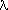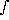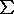# Rayleigh Scattering: Optical Depth

The average scattering cross section per particle is given by() = (12852)/(34) · [ (6 + 3)/(6 - 7) ]= (n - 1)/(2N0)(n2 - 1)/(4N0),   for n near unity

where, n is the index of refraction, N0 is the number of molecules per unit volume at standard temperature and pressure ( i.e., conditions of index of refraction),is the depolarization factor.

The optical depth can be related to the ``thickness'' of the atmosphere. If Z is the thickness in Km-amagats then:ray() =N(z) ·() dz() ·N(z) · dz = Z · N0 ·()

The index of refraction has a wavelength dependence. This is usually represented by two constants, A and B, as follows:

(n-1) = A · (1 + B/2),in µm

(n-1)2 = A2 · (1 + 2 B/2 + B2/4)ray = Z a0/4 · (1 + a1/2 + a2/4)   where,

a0 = (323)/(3 N02) · [(6 + 3)/(6 - 7) ] ·qi · A2

a1 =qi · 2 B,   and   a2 =qi · B2ray(H2) = Z · 2.19 · 10-4/4 · ( 1 + 0.0157248/2 + 0.0001978/4 ),in µm

The wavelength dependence in the VIAMP code is taken from Dalgarno and Williams (ApJ, 1962). This equation is similar to the equation above, however, it differs in the8 term.ray(H2) = (8.14 · 10-13/4 + 1.28 · 10-6/6 + 1.61/8) cm2,in Å

The optical depth per Km-amagat of hydrogen, denoted by1(H2), is then1(H2) = 2.687 · 1024 ·ray(H2) = 2.687 (8.14 · 1011/4 + 1.28 · 1018/6 + 1.61 · 1024/8),in Å1(H2) = 2.687 (8.14 · 10-21 f4 + 1.28 · 10-30 f6 + 1.61 · 10-40 f8),   f = 108/andin Å

And the total optical depth for a mixture of gases is given asray =1(H2) ·Zi · [ (ni - 1)2 / (nH2 - 1)2 ]

Note that this formulation always uses the wavelength dependence of hydrogen, even if other gases are used.

(n-1) = A · (1 + B/2),in µm

ray = (n-1)2/(nH2 - 1)2

 ray n A(10-5) B(10-3)(10-24)a0µm4/Km air 4.4459 1.0002918 28.71 5.67 3.40 0.031 10.7E-4 H2 1.0000 1.0001384 13.58 7.52 1.61 0.02 2.35E-4 He 0.0641 1.0000350 3.48 2.30 O2 3.8634 1.000272 26.63 5.07 0.054 N2 4.6035 1.000297 29.06 7.7 3.44 0.030 10.9E-4 H2O 3.3690 1.000254 CO2 10.5611 1.0004498 43.9 6.4 0.09 CO 5.8247 1.000334 32.7 8.1 NH3 7.3427 1.000375 37.0 12.0 NO 4.6035 1.000297 28.9 7.4 CH4 10.1509 1.000441

The depth of penetration of Rayleigh scattering is computed for various objects using the data above. A summary of the characteristics of the plots in Figure 9 is given below (g is gravity in cm/s2, µ = molecular weight in gm/mole, Z = KM-amagats per Bar).

 wave(µm): 0.1 0.2 0.264 0.3 0.4 0.5(P0): 209.57 6.95 2.05 1.19 0.36 0.14 P(=1): 4.8 145.7 493.2 851 2834.7 7094.5

 wave(µm): 0.1 0.2 0.264 0.3 0.4 0.5(P0): 299.82 8.62 2.47 1.42 0.42 0.17 P(=1): 3.3 116.1 405.2 706.3 2390.8 6030.4

 wave(µm): 0.1 0.2 0.264 0.3 0.4 0.5(P0): 754.31 21.68 6.21 3.56 1.05 0.42 P(=1): 1.3 46.1 161 280.7 950.3 2396.9

 wave(µm): 0.1 0.2 0.264 0.3 0.4 0.5(P0): 5378.65 152.59 43.58 24.98 7.37 2.92 P(=1): 0.3 9.8 34.4 60.1 203.6 513.9

The gas continuum absorption contains a term which is supposed to represent the effect of Raman scattering due to the1 vibration of H2 at 4161 cm-1.
Ref.: Belton et al. (1971). Atm. of Uranus. ApJ. 164, 191-209raman = 0.0208 ·ray